# Physics Homework Help With Motion With Constant Acceleration

## Motion With Constant Acceleration

In this section, we are going to derive relations among displacement, velocity, acceleration and time.
We know that,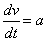On integrating both sides, we get
v = at + c1 where c1 = constant of integration

If v0 be the initial velocity of the particle then at t = 0, v = v0. This implies that c1 = v0
v = v0 + at … (1)
Also we know that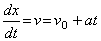or dx = (v0 + at)dt

On integrating, we get
òdx = òv0dt + òatdt
x = v0t +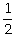at2 + c2 where c2 = constant

If x0 be the initial position of the particle, then at t = 0; x = x0. This implies that c2 = x0
x = x0 + v0t +at2 … (2)

Eliminating t from equations (1) and (2), we get
v2 =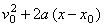… (3)

The equation (2) may be rewritten as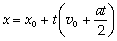From equation (1), we have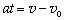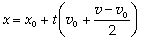thus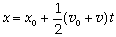… (4)

The equations (1) to (4) are called the equations of kinematics in the direction along the x-axis.
The equations of kinematics are summarized as
v = v0 + at (1)
x = x0 + v0t +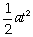(2)
v2 =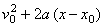(3)
x = x0 +(v0 + v)t (4)

where x0 = initial position coordinate; x = Final position coordinate
v = final velocity; a = acceleration (constant)

### Homework Help For Motion with Constant Acceleration

assignmenthelp.net provides best Online Assignment Help service in Physics for all standards. Our Tutor provide their high quality and optimized Tutorial help to fulfill all kind of need of Students.